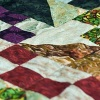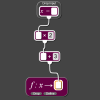# Resources tagged with: Factors and multiples

Filter by: Content type:
Age range:
Challenge level:

### There are 106 results

Broad Topics > Properties of Numbers > Factors and multiples### Common Divisor

##### Age 14 to 16Challenge Level

Find the largest integer which divides every member of the following sequence: 1^5-1, 2^5-2, 3^5-3, ... n^5-n.### Data Chunks

##### Age 14 to 16Challenge Level

Data is sent in chunks of two different sizes - a yellow chunk has 5 characters and a blue chunk has 9 characters. A data slot of size 31 cannot be exactly filled with a combination of yellow and. . . .### Just Repeat

##### Age 11 to 14Challenge Level

Think of any three-digit number. Repeat the digits. The 6-digit number that you end up with is divisible by 91. Is this a coincidence?### Mathematical Swimmer

##### Age 11 to 14Challenge Level

Can you work out how many lengths I swim each day?### Funny Factorisation

##### Age 11 to 16Challenge Level

Using the digits 1 to 9, the number 4396 can be written as the product of two numbers. Can you find the factors?##### Age 11 to 14Challenge Level

List any 3 numbers. It is always possible to find a subset of adjacent numbers that add up to a multiple of 3. Can you explain why and prove it?### Alison's Quilt

##### Age 11 to 14Challenge Level

Nine squares are fitted together to form a rectangle. Can you find its dimensions?##### Age 11 to 14Challenge Level

Make a set of numbers that use all the digits from 1 to 9, once and once only. Add them up. The result is divisible by 9. Add each of the digits in the new number. What is their sum? Now try some. . . .### Why 24?

##### Age 14 to 16Challenge Level

Take any prime number greater than 3 , square it and subtract one. Working on the building blocks will help you to explain what is special about your results.### Even So

##### Age 11 to 14Challenge Level

Find some triples of whole numbers a, b and c such that a^2 + b^2 + c^2 is a multiple of 4. Is it necessarily the case that a, b and c must all be even? If so, can you explain why?### Divisibility Tests

##### Age 11 to 16

This article explains various divisibility rules and why they work. An article to read with pencil and paper handy.### What a Joke

##### Age 14 to 16Challenge Level

Each letter represents a different positive digit AHHAAH / JOKE = HA What are the values of each of the letters?### Really Mr. Bond

##### Age 14 to 16Challenge Level

115^2 = (110 x 120) + 25, that is 13225 895^2 = (890 x 900) + 25, that is 801025 Can you explain what is happening and generalise?### Inclusion Exclusion

##### Age 11 to 14Challenge Level

How many integers between 1 and 1200 are NOT multiples of any of the numbers 2, 3 or 5?### Number Rules - OK

##### Age 14 to 16Challenge Level

Can you produce convincing arguments that a selection of statements about numbers are true?### Remainder

##### Age 11 to 14Challenge Level

What is the remainder when 2^2002 is divided by 7? What happens with different powers of 2?### Two Much

##### Age 11 to 14Challenge Level

Explain why the arithmetic sequence 1, 14, 27, 40, ... contains many terms of the form 222...2 where only the digit 2 appears.### Counting Cogs

##### Age 7 to 14Challenge Level

Which pairs of cogs let the coloured tooth touch every tooth on the other cog? Which pairs do not let this happen? Why?### Exploring Simple Mappings

##### Age 11 to 14Challenge Level

Explore the relationship between simple linear functions and their graphs.### Multiplication Magic

##### Age 14 to 16Challenge Level

Given any 3 digit number you can use the given digits and name another number which is divisible by 37 (e.g. given 628 you say 628371 is divisible by 37 because you know that 6+3 = 2+7 = 8+1 = 9). . . .### Satisfying Statements

##### Age 11 to 14Challenge Level

Can you find any two-digit numbers that satisfy all of these statements?### A Biggy

##### Age 14 to 16Challenge Level

Find the smallest positive integer N such that N/2 is a perfect cube, N/3 is a perfect fifth power and N/5 is a perfect seventh power.### Big Powers

##### Age 11 to 16Challenge Level

Three people chose this as a favourite problem. It is the sort of problem that needs thinking time - but once the connection is made it gives access to many similar ideas.### Three Times Seven

##### Age 11 to 14Challenge Level

A three digit number abc is always divisible by 7 when 2a+3b+c is divisible by 7. Why?### Counting Factors

##### Age 11 to 14Challenge Level

Is there an efficient way to work out how many factors a large number has?### Number Families

##### Age 11 to 14Challenge Level

How many different number families can you find?### What Numbers Can We Make Now?

##### Age 11 to 14Challenge Level

Imagine we have four bags containing numbers from a sequence. What numbers can we make now?### Repeaters

##### Age 11 to 14Challenge Level

Choose any 3 digits and make a 6 digit number by repeating the 3 digits in the same order (e.g. 594594). Explain why whatever digits you choose the number will always be divisible by 7, 11 and 13.### Mod 3

##### Age 14 to 16Challenge Level

Prove that if a^2+b^2 is a multiple of 3 then both a and b are multiples of 3.### Hot Pursuit

##### Age 11 to 14Challenge Level

I added together the first 'n' positive integers and found that my answer was a 3 digit number in which all the digits were the same...### Dozens

##### Age 7 to 14Challenge Level

Can you select the missing digit(s) to find the largest multiple?### Factor Track

##### Age 7 to 14Challenge Level

Factor track is not a race but a game of skill. The idea is to go round the track in as few moves as possible, keeping to the rules.### Can You Find a Perfect Number?

##### Age 7 to 14### Eminit

##### Age 11 to 14Challenge Level

The number 8888...88M9999...99 is divisible by 7 and it starts with the digit 8 repeated 50 times and ends with the digit 9 repeated 50 times. What is the value of the digit M?### Thirty Six Exactly

##### Age 11 to 14Challenge Level

The number 12 = 2^2 × 3 has 6 factors. What is the smallest natural number with exactly 36 factors?### American Billions

##### Age 11 to 14Challenge Level

Play the divisibility game to create numbers in which the first two digits make a number divisible by 2, the first three digits make a number divisible by 3...##### Age 11 to 14Challenge Level

Great Granddad is very proud of his telegram from the Queen congratulating him on his hundredth birthday and he has friends who are even older than he is... When was he born?### Flow Chart

##### Age 11 to 14Challenge Level

The flow chart requires two numbers, M and N. Select several values for M and try to establish what the flow chart does.### Factoring Factorials

##### Age 11 to 14Challenge Level

Find the highest power of 11 that will divide into 1000! exactly.### Product Sudoku

##### Age 11 to 16Challenge Level

The clues for this Sudoku are the product of the numbers in adjacent squares.### Different by One

##### Age 14 to 16Challenge Level

Can you make lines of Cuisenaire rods that differ by 1?### The Remainders Game

##### Age 7 to 14Challenge Level

Play this game and see if you can figure out the computer's chosen number.### What Numbers Can We Make?

##### Age 11 to 14Challenge Level

Imagine we have four bags containing a large number of 1s, 4s, 7s and 10s. What numbers can we make?### Missing Multipliers

##### Age 7 to 14Challenge Level

What is the smallest number of answers you need to reveal in order to work out the missing headers?### Sieve of Eratosthenes

##### Age 11 to 14Challenge Level

Follow this recipe for sieving numbers and see what interesting patterns emerge.### Transposition Cipher

##### Age 11 to 16Challenge Level

Can you work out what size grid you need to read our secret message?### Got it for Two

##### Age 7 to 14Challenge Level

Got It game for an adult and child. How can you play so that you know you will always win?### Multiples Sudoku

##### Age 11 to 14Challenge Level

Each clue in this Sudoku is the product of the two numbers in adjacent cells.### Gabriel's Problem

##### Age 11 to 14Challenge Level

Gabriel multiplied together some numbers and then erased them. Can you figure out where each number was?### A First Product Sudoku

##### Age 7 to 14Challenge Level

Given the products of adjacent cells, can you complete this Sudoku?